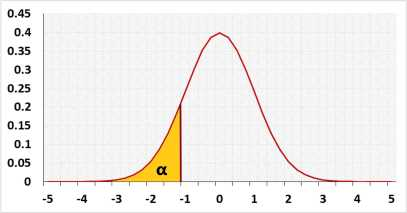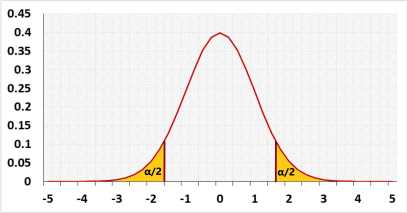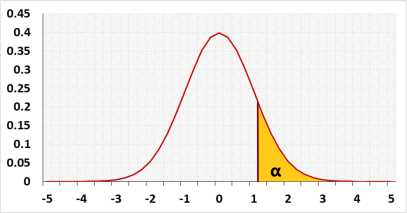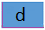﻿One sample proportion test

# One Sample proportion Test

## Proportion z Test or Binomial test

The binomial test is an exact test base on the binomial distribution while the proportion z-test uses the normal approximation.
For a small sample please use the binomial test.

Hypotheses
H0: p = P0
H1: p < > P0
Test statisticNormal distributionTest parameters
The default is two tailed test.
example digits=2: (0.001234 => 0.0012)
Significant level (0-1), maximum chance allowed rejecting H0 while H0 is correct (Type1 Error)
continuity correction from binomial distribution to the normal distribution, the default is True. relevant only to z-test
The test is expected to identify this effect. If one exists, H0 will be rejected. h effects examples( 0.2 - small effect, 0.5 - medium effect, 0.8 - large effect). more
Expected difference, usually zero
You may calculate h based on the expected proportions:

### Calcualate h effect size

if real p equals this value, you want the test to be significance
the expected p based on history or other researches

Sample data

The population's name
sample1's proportion or number of successes
Sample size
Expected proportion

## Information

Target: To check if the difference between the probability of succees (p) of two groups (populations) is significant, using sample data

## AssumptionsBinomial distribution - the probability for event within each group is identicalExpected difference d between the populations's average is known
Required Sample Datap the sample probabilities or x the number of successesn - Sample size

## R Code

The following R code should produce the same results:

The prop.test uses the Chi-squared statistic with one degree of freedom, which is exactly as using the Z test: z22(1)